• 2020-02-10 15:42:29

在做数据分析的过程中。我们往往是–理解需求–获取数据–清洗数据–简单描述统计–统计型分析报告；
其实到这里还没完。如果我们还需要深入探索数据的价值，那么，单变量的分布检验–探索变量间的关系–建立关系模型–评估–总结等。
接下来就来看看数据分析的其中重要的一环–探索变量间的关系。

# 探索变量之间的关系

探索数据变量之间是否存在某种关系/关联。大致步骤有：

• 变量的类型：类别型/数值型
• 可视化给出可能的方向：散点图、箱型图、直方图、…
• 需建立更严格的分析方式：假设检验。假设变量间存在某种函数/逻辑等关联关系，进行检验。

# 一、准备工具和数据

## 1.1、工具：我们用python3

通常我们把用于做数据分析的几个库直接先导入，基本是固定导入使用。

import numpy as np               #科学计算基础库，多维数组对象ndarray
import pandas as pd              #数据处理库，DataFrame（二维数组）
import matplotlibpython3 python
更多相关内容
• 相关关系变量之间存在的不确定的数量关系，称为相关关系。 相关分析就是对两个变量之间线性关系的描述与度量，要解决的问题如下： 变量之间是否存在关系？ 如果存在关系，它们之间是什么关系变量之间关系...

本章内容：

1. 判断两个变量间是否有相关关系，且关系强度如何？
2. 如何建立一元线性回归模型，且模型效果如何？
3. 如何利用回归方程进行预测？
4. 为什么要进行残差分析，及如何进行分析？

索引
📌 专业名词
🔑 公式记忆
📖 摘抄
☑️ 有序事项

# 11.1 变量间是否有相关关系，且关系强度如何？

### 11.1.2 相关关系的描述与测量

📌 相关关系：变量之间存在的不确定的数量关系，称为相关关系。

📖 相关分析就是对两个变量之间线性关系的描述与度量，要解决的问题如下：

1. 变量之间是否存在关系？
2. 如果存在关系，它们之间是什么关系？
3. 变量之间的关系强度如何？
4. 样本反映的变量之间的关系能否代表总体变量之间的关系？

进行相关分析时，对总体主要有以下两个假定：

1. 两个变量都是线性关系
2. 两个变量都是随机变量

📖 如何判断变量之间的相关形态？

1. 散点图——用于判断是否存在关系、什么关系

2. 相关系数——用于判断关系强度如何？

🔑 相关关系计算公式
若相关系数是根据总体全部数据计算的，称为总体相关系数，记为 ρ \rho ； 若是根据样本数据计算的，称为样本相关系数，记为 r r ，计算公式如下：

r = n ∑ x y − ∑ x ∑ y n ∑ x 2 − ( ∑ x ) 2 ⋅ n ∑ y 2 − ( ∑ y ) 2 r=\frac{ n\sum{xy}-\sum{x}\sum{y} }{ \sqrt{ n\sum{x^2}-(\sum{x})^2 }\cdot{ \sqrt{ n\sum{y^2}-(\sum{y})^2 } } }

上式计算得出的相关系数也称为线性相关系数，或皮尔逊（Pearson）相关系数。

Excel中，计算两组数据的相关系数，公式为：CORREL(array1,array2)
【工具】-【数据分析】

📖 相关系数的性质：

1. 取值范围是[-1,1]。 如果 0 < r ≤ 1 0<r≤1 ，表明x与y之间存在正线性相关关系； 如果 − 1 ≤ r < 0 -1≤r<0 ，表明x与y之间存在负线性相关关系； 如果 r = 1 r=1 ,表明x与y之间存在完全正线性相关关系； 如果 r = − 1 r=-1 ,表明x与y之间存在完全负线性相关关系； 如果 r = 1 r=1 ,表明x与y之间不存在相关关系；
2. 对称性，即 r x y = r y x r_{xy}=r_{yx}
3. r的数值大小与x和y的原点及尺度无关。改变x和y的数据原点及计量尺度，并不改变数值大小。
4. r仅仅是x与y之间线性关系的一个度量，不能用于描述非线性关系。即 r = 0 r=0 只能表示变量之间不存在线性相关关系，不说明变量之间没有任何相关关系。
5. r不意味着x与y一定有因果关系。

🔑 r的大小与相关程度划分

∣ r ∣ ≥ 0.8 |r|≥0.8 时，可视为高度相关；
0.5 ≤ ∣ r ∣ < 0.8 0.5≤|r|<0.8 时，可视为中度相关；
0.3 ≤ ∣ r ∣ < 0.5 0.3≤|r|<0.5 时，可视为低度相关；
∣ r ∣ < 0.3 |r|<0.3 时，可视为相关性极弱，可视为不相关；

但这种解释必须建立在对相关系数的显著性进行检验的基础之上！

### 11.1.3 相关关系的显著性检验

📖 为什么要进行显著性检验？
因为总体相关系数 ρ ρ 通常未知，一般使用样本相关系数 r r 来代替总体相关系数，但是样本相关系数受到抽样数据的影响，所以需要对样本相关系数说明总体的相关程度，即进行显著性检验

📖 r的抽样分布
样本相关系数r的显著性与r的抽样分布相关；r的抽样分布随着总体相关系数和样本量的大小而变化。

当样本数据来自正态总体时，随着n的增大，r的抽样分布趋于正态分布。
当总体相关系数接近0时，r趋于正态分布的趋势非常明显；反之r的抽样分布呈现一定的偏态。
ρ ρ 为较大的正值时， r r 呈现左偏分布；
ρ ρ 为较大的负值时，r呈现右偏分布。 只有当 ρ ρ 接近于0，而样本量n很大时，才能认为r是接近于正态分布的随机变量。

☑️ r的显著性检验

1. 提出假设

H 0 : ρ = 0 ; H 1 : ρ ≠ 0 H_0:ρ=0;H_1:ρ≠0

1. 计算检验的统计量：t统计量 t = ∣ r ∣ n − 2 1 − r 2 ∼ t ( n − 1 ) t=|r|\sqrt{\frac{ n-2 }{ 1-r^2 }}\sim{t(n-1)} 其自由度为： n − 2 n-2

2. 进行决策 根据给定的显著性水平 α \alpha 和自由度，计算 t α / 2 t_{\alpha/2} 。 若 ∣ t ∣ > t α / 2 |t|>t_{α/2} ，则拒绝原假设 H 0 H_0 ，表明总体的两个变量之间存在显著的线性关系。

# 11.2 如何建立一元线性回归模型，且进行模型检验？

📖 相关分析的目的在于考察变量之间的关系强度；
回归分析的目的在于考察变量之间的数量关系，并通过一定的数学表达式将这种关系描述出来，进而确定一个或几个变量的变化对另一个特定变量的影响程度。

回归分析解决的问题：

1. 从样本数据出发，确定变量之间的数学关系式；
2. 对这些关系式的可信程度进行各种统计检验，并从影响某一特定变量的诸多变量中找出哪些变量的影响是显著的，哪些是不显著的；
3. 利用所求的关系式，根据一个或几个变量的取值来估计或预测另一个特定变量的取值，并给出这种估计或预测的可靠程度。（这部分内容实际放在11.3章来讲解）

### 11.2.1 一元线性回归

📌 常见专业名词解释
因变量
被预测或被解释的变量称为因变量，用y表示。

自变量
用来预测或解释因变量的一个或多个变量称为自变量，用x表示。

一元回归
当回归中只涉及一个自变量时，称为一元回归。

一元线性回归
若因变量x和自变量y之间为线性关系，称为一元线性回归。

📖 本章所讨论的回归方法对于自变量是预先固定的、自变量是随机的情况都使用；
因为固定自变量的情况比较容易描述，因此下面主要讲述固定自变量的回归问题。

📌 回归模型

对于具有线性关系的两个变量，可以用一个线性方程来表示它们之间的关系。 描述因变量y如何依赖于自变量x和误差项 ε ε 的方程，称为回归模型。

📌 理论回归模型
对于只涉及一个自变量的一元线性回归模型可表示为：

y = β 0 + β 1 x + ε y=β_0+β_1x+\varepsilon

其中 β 0 + β 1 x β_0+β_1x 反映了由于x的变化，引起y的线性变化； ε \varepsilon 是误差项的随机变量，反映了线性关系之外的随机因素对y的影响。 β 0 、 β 1 \beta_0、\beta_1 称为模型的参数。

📖 理论回归模型的假定

①因变量y与自变量x之间具有线性关系；

②在重复抽样中，自变量x的取值是固定的，即假定x是非随机的；

③误差项 ε \varepsilon 是一个期望值为0的随机变量，即 E ( ε ) = 0 E(\varepsilon)=0

④对于所有的x值， ε \varepsilon 的方差 σ 2 \sigma^2 都相同；因为误差与x、y都无关。

⑤误差项 ε \varepsilon 是一个服从正态分布的随机变量，且独立，即 ε ∼ N ( 0 , σ 2 ) \varepsilon\sim{N(0,σ^2)}
E(y)的值随着x的不同而变化，但无论x怎么变化， ε \varepsilon 和y的概率分布都是正态分布，并且具有相同的方差。

📌 回归方程
描述因变量y的期望值如何依赖于自变量x的方程，称为回归方程。
一元线性回归方程也称为直线回归方程，公式如下：

E ( y ) = β 0 + β 1 x E(y)=β_0+β_1x

β 0 \beta_0 为截距， β 1 \beta_1 为斜率。

📌 估计的回归方程
由于总体是未知的，所以采用样本统计量 β 0 ^ \hat{\beta_0} β 1 ^ \hat{\beta_1} 代替回归方程中的未知参数 β 0 \beta_0 β 1 \beta_1 ，得到估计的回归方程。

一元线性回归下，估计的回归方程为：

y ^ = β ^ 0 + β ^ 1 x \hat{y}=\hat{β}_0+\hat{β}_1x

### 11.2.2 参数的最小二乘估计

📖 最小二乘法是确定直线代表最强代表性的方法。

因为描述x,y的n对观测值的直线，有多条，所以需要找到一种方法，来确定最佳代表两个变量之间关系的直线。

📌 最小二乘法
代表两个变量之间关系的标准之一：该直线距离各个观测点的距离最近。
通过使用因变量的观测值 y i y_i ，和估计值 y ^ i \hat{y}_i 之间的离差平方和来估计参数 β 0 \beta_0 β 1 \beta_1 的方法，称为最小二乘法，也称为最小平方法。

📖 优势如下：

①根据最小二乘法得到的回归直线能使离差平方和达到最小，虽然这并不能保证它就是拟合数据的最佳直线，但这毕竟是一条与数据拟合良好的直线应有的性质；

②由最小二乘法求得的回归直线可知β0和β1的估计量的抽样分布；

③在某些条件下β0和β1的最小二乘估计量同其他估计量相比，其抽样分布具有较小的标准差。

🔑 求解 β 0 ^ \hat{\beta_0} β 1 ^ \hat{\beta_1}

构建最小二乘法公式：

∑ ( y i − y ^ i ) 2 = ∑ ( y i − β ^ 0 − β ^ 1 x i ) 2 \sum{(y_i-\hat{y}_i)}^2 =\sum{ ( y_i-\hat{β}_0-\hat{β}_1x_i )^2 }

根据最小二乘法原理，要求得上式最小值。根据微积分的极限定理，令 Q = ∑ ( y i − y ^ i ) 2 Q=\sum{(y_i-\hat{y}_i)}^2 ，即对Q求相应于 β 0 ^ \hat{\beta_0} β 1 ^ \hat{\beta_1} 的偏导数，并令其等于0。 \ 求解 β 0 ^ \hat{\beta_0} β 1 ^ \hat{\beta_1} 公式：

{ ∂ Q ∂ β 0 ∣ β 0 = β ^ 0 = − 2 ∑ i = 1 n ( y i − β ^ 0 − β ^ 1 x i ) 2 = 0 ∂ Q ∂ β 1 ∣ β 1 = β ^ 1 = − 2 ∑ i = 1 n x i ( y i − β ^ 0 − β ^ 1 x i ) 2 = 0 \left\{ \begin{aligned} \frac{ \partial{Q} }{ \partial{\beta_0} }|_{\beta_0=\hat{\beta}0} & = -2\sum_{i=1}^n ( y_i-\hat{β}_0-\hat{β}1x_i )^2 =0\\ \frac{ \partial{Q} }{ \partial{\beta_1} }|_{\beta_1=\hat{\beta}1} & = -2\sum_{i=1}^n x_i( y_i-\hat{β}_0-\hat{β}_1x_i )^2 =0 \end{aligned} \right.

{ β ^ 1 = n ∑ i = 1 n x i y i − ∑ i = 1 n x i ∑ i = 1 n y i n ∑ i = 1 n x i 2 − ( ∑ i = 1 n x i ) 2 β ^ 0 = y ˉ − β ^ 1 x ˉ \left\{ \begin{aligned} \hat{\beta}1 & = \frac{ \displaystyle n\sum_{i=1}^nx_iy_i-\sum_{i=1}^nx_i \sum_{i=1}^ny_i }{ \displaystyle n\sum_{i=1}^nx_i^2-( \sum_{i=1}^nx_i)^2 } \\ \hat{\beta}_0 & = \bar{y}-\hat{\beta}_1\bar{x} \end{aligned} \right.

excel中，求解一元线性回归方程的方式为：

【工具】-【回归】

### 11.2.3 回归直线的拟合优度

📌 拟合优度 回归直线与各观测点的接近程度称为回归直线对数据的拟合优度。 各观测点越是紧密围绕直线，说明一元线性回归模型对观测数据的拟合程度越好。

🔑 判定系数- R 2 R^2
判定系数是对估计的回归方程拟合优度的度量，其公式如下：

R 2 = S S R S S T = ∑ ( y ^ i − y ˉ i ) 2 ∑ ( y i − y ˉ i ) 2 R^2=\frac{SSR}{SST} =\frac{ \sum{(\hat{y}_i-\bar{y}_i)}^2 }{ \sum{(y_i-\bar{y}_i)}^2 }

判定系数就是：回归平方和/总平方和；判定系数越接近1，说明回归直线的拟合效果越好；反之。

判定系数的实际意义：在y取值的变动中，有 R 2 R^2 （这是个百分比）的部分可以由x与y之间的线性关系来解释；即y中有 R 2 R^2 是由x决定的。

📌 估计标准误差
度量各个实际观测点在直线周围的散布状况的一个统计量，用来说明实际观测值与回归估计值之间的差异程度。

🔑 估计标准误差的公式为：

s e = ∑ ( y i − y ^ i ) 2 n − 2 = S S E n − 2 = M S E s_e=\sqrt{\frac{ \sum{(y_i-\hat{y}_i)^2} }{ n-2 }} =\sqrt{\frac{ SSE }{ n-2 }} =\sqrt{MSE}

可以看做在排除了x对y的线性影响后，y随机波动大小的一个估计量。 反映了用估计的回归方程预测因变量y时预测误差的大小。

和判定系数 R 2 R^2 的差异：
判定系数是百分比，不是实际的数值， 1 − R 2 1-R^2 在一定程度上也衡量了差异程度，但是不是具体的差异值；
估计标准误差是具体的差异值。

### 11.2.4 显著性检验

📖 回归分析中的显著性检验包括2部分：

线性关系的检验_F统计量
回归系数的检验_t统计量

☑️ 线性关系检验 _F统计量
检验自变量x和因变量y之间的线性关系是否显著，即它们之间能否使用一元线性回归模型来表示。

1. 构造统计量
F = S S R / 1 S S E / ( n − 2 ) = M S R M S E ∼ F ( 1 , n − 2 ) F=\frac{ SSR/1 }{ SSE/(n-2) } =\frac{MSR}{MSE}\sim{F(1,n-2)}
即：
F = 回 归 平 方 和 / 自 由 度 ： k ( 自 变 量 的 个 数 ) 残 差 平 方 和 / 自 由 度 ： n − k − 1 = 均 方 回 归 均 方 残 差 ∼ F ( 1 , n − 2 ) F=\frac{ 回归平方和/自由度：k(自变量的个数) }{ 残差平方和/自由度：n-k-1 } =\frac{ 均方回归 }{ 均方残差 }\sim{F(1,n-2)}

2. 提出假设： H 0 : β 1 = 0 H_0:\beta_1=0 ，两个变量之间的线性关系不显著，即没有线性关系。

3. 计算统计量F

4. 做出决策
F > F α F>F_\alpha ，拒绝原假设，两个变量之间有线性关系；
F < F α F<F_\alpha ，接受原假设，两个变量之间有线性关系。

☑️ 回归系数检验 _t统计量
检验自变量对因变量的影响是否显著，也是检查两个变量之间有没有线性关系的。
如果 β 1 = 0 \beta_1=0 ，那么两个变量之间没有线性关系；
如果 β 1 ≠ 0 \beta_1≠0 ，那么两个变量之间有线性关系。

为什么不能直接计算 β 1 \beta_1 来判断两个变量的线性关系呢？
因为总体的数据量是未知的，所以不能计算 β 1 \beta_1 ，只能通过样本量得到 β ^ 1 \hat{\beta}_1 ；通过 β ^ 1 \hat{\beta}_1 的统计量检验，才能判断总体 β 1 \beta_1 的情况。

1. 构建统计量t
t = β ^ 1 − β 1 s β ^ 1 ∼ t ( n − 2 ) t=\frac{ \hat{\beta}1-\beta_1 }{ s{\hat{\beta}_1} }\sim{t(n-2)}
自由度为：n-2
其中 s β ^ 1 s_{\hat{\beta}_1} 计算公式如下：
s β ^ 1 = s e ∑ x i 2 − 1 n ( ∑ x i ) 2 s_{\hat{\beta}_1}=\frac{ s_e }{ \sqrt{ \sum{x_i^2}-\frac{1}{n}(\sum{x_i})^2 } }
s e = ∑ ( y i − y ^ i ) 2 n − 2 = S S E n − 2 = M S E s_e=\sqrt{\frac{ \sum{(y_i-\hat{y}_i)^2} }{ n-2 }} =\sqrt{\frac{ SSE }{ n-2 }} =\sqrt{MSE}
其中， s e s_e σ \sigma 的估计量，称为估计标准误差；因为 σ \sigma 通常未知，所以用 s β ^ 1 s_{\hat{\beta}1} 作为 σ β ^ 1 \sigma{{\hat{\beta}_1}} 的估计量。

2. 提出检验： H 0 : β 1 = 0 H_0:\beta_1=0 ，两个变量之间的线性关系不显著，即没有线性关系。

3. 计算检验统计量t

4. 做出决策
∣ t ∣ > t α / 2 |t|>t_{\alpha/2} ，拒绝原假设，两个变量之间有线性关系；
∣ t ∣ < t α / 2 |t|<t_{\alpha/2} ，接受原假设，两个变量之间没有线性关系。

📖 excel数据分析中，其他参数的计算公式：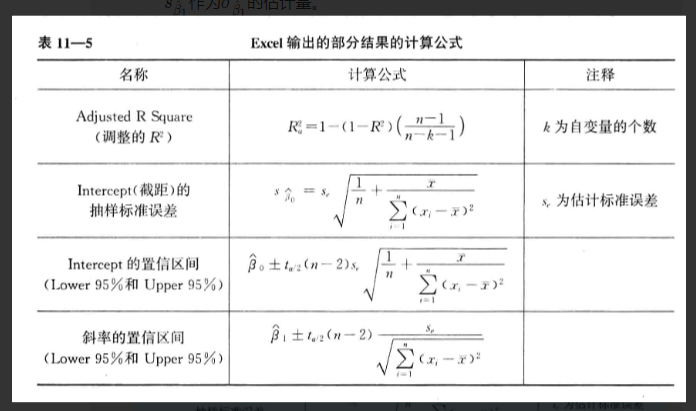### 11.2.5 回归分析结果的评价

☑️ 如何判断一元线性回归模型的效果

（1）看 β ^ 1 \hat{β}_1 ：所估计的回归系数 β ^ 1 \hat{β}_1 的符号是否与理论或事先预期相一致；

（2）看t检验：判断回归系数是否显著。如果理论上认为y与x之间的关系不仅是正的，而且是统计上显著的，那么所建立的回归方程也应该如此；

（3）看 R 2 R^2 ：可以用判定系数 R 2 R^2 来回答回归模型在多大程度上解释了因变量y取值的差异；

（4）看F检验：考察关于误差项ε的正态性假定是否成立。

# 11.3 如何利用回归方程进行预测？

### 11.3.1 点估计

📖 利用估计的回归方程，对于x的一个特定值x0，求出y的一个估计值就是点估计。

点估计可分为两种：
一是平均值的点估计；
二是个别值的点估计。

在点估计的条件下，对于同一个x0，平均值的点估计和个别值的点估计的结果是一样的，但在区间估计中则有所不同。

### 11.3.2 区间估计

📖 利用估计的回归方程，对于x的一个特定值x0，求出y的一个估计值的区间就是区间估计。

区间估计也有两种类型：
一是置信区间估计，它是对x的一个给定值x0，求出y的平均值的估计区间，这一区间称为置信区间；
二是预测区间估计，它是对x的一个给定值x0，求出y的一个个别值的估计区间，这一区间称为预测区间。

🔑 置信区间估计
通过 y ^ 0 \hat{y}_0 估计 E ( y 0 ) E(y_0) 的区间，需要知道 y ^ 0 \hat{y}_0 的标准差，用 s y ^ 0 s_{\hat{y}_0} 表示 y ^ 0 \hat{y}_0 的标准差的估计量。

y ^ 0 \hat{y}_0 的置信区间如下：

y ^ 0 ± t α / 2 s e 1 n + ( x 0 − x ˉ ) 2 ∑ i = 1 n ( x i − x ˉ ) 2 \hat{y}0\pm{t}{\alpha/2}s_e \sqrt{ \frac{1}{n} +\frac{ (x_0-\bar{x})^2 }{\displaystyle \sum_{i=1}^n(x_i-\bar{x})^2 } }

🔑 预测区间估计

y ^ 0 ± t α / 2 s e 1 + 1 n + ( x 0 − x ˉ ) 2 ∑ i = 1 n ( x i − x ˉ ) 2 \hat{y}0\pm{t}{\alpha/2}s_e \sqrt{ 1+ \frac{1}{n} +\frac{ (x_0-\bar{x})^2 }{\displaystyle \sum_{i=1}^n(x_i-\bar{x})^2 } }

对比预测区间估计、置信区间估计的公式，预测区间估计的公式根号内多了一个1。
可见，预测区间要比置信区间更宽。

📖 注意：在利用回归方程进行估计或预测时，不要用样本数据最大最小值之外的x值去预测相对应的y值，结果会比较不理想。

# 11.4 为什么要进行残差分析，及如何分析？

### 11.4.1 残差与残差图

📖 一元相信回归模型中，假定 ε \varepsilon 是期望值为0、方差相等且服从正态分布的一个随机变量。

如果假定不满足，则检验、估计和预测则可能站不住脚，所以需要对 ε \varepsilon 的假定是否成立进行判断，方法之一就是残差分析。

📌 残差
因变量的观测值 y i y_i 与根据估计的回归方程求出的预测值 y ^ i \hat{y}_i 之差，用 e e 表示。
反映了用估计的回归方程去预测 y i y_i 引起的误差，公式如下：

e i = y i − y ^ i e_i=y_i-\hat{y}_i

📌 残差图
用于判断 ε \varepsilon 的假定是否成立；其中x为横轴， e e 为纵轴。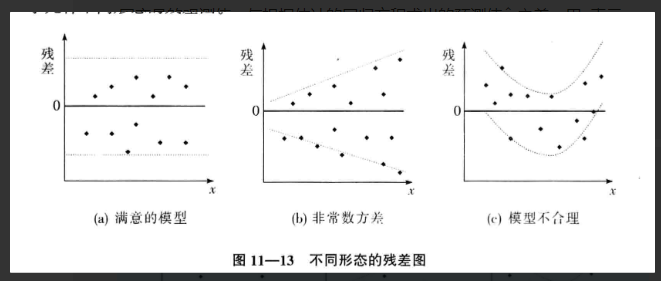### 11.4.2 标准化残差

标准化残差
用于判断 ε \varepsilon 的假定是否成立；是残差除以它的标准差后得到的数值，用 z e z_e 表示，公式如下：
z e i = e i s e = y i − y ^ i s e z_{e_i}=\frac{e_i}{s_e}=\frac{y_i-\hat{y}_i}{s_e}

如果误差项 ε ε 服从正态分布这一假定成立，那么标准化残差的分布也应服从正态分布。
因此，在标准化残差图中，大约有95%的标准化残差在-2～+2之间。

书籍：《统计学（第六版）》
书籍作者：贾俊平

内容思维导图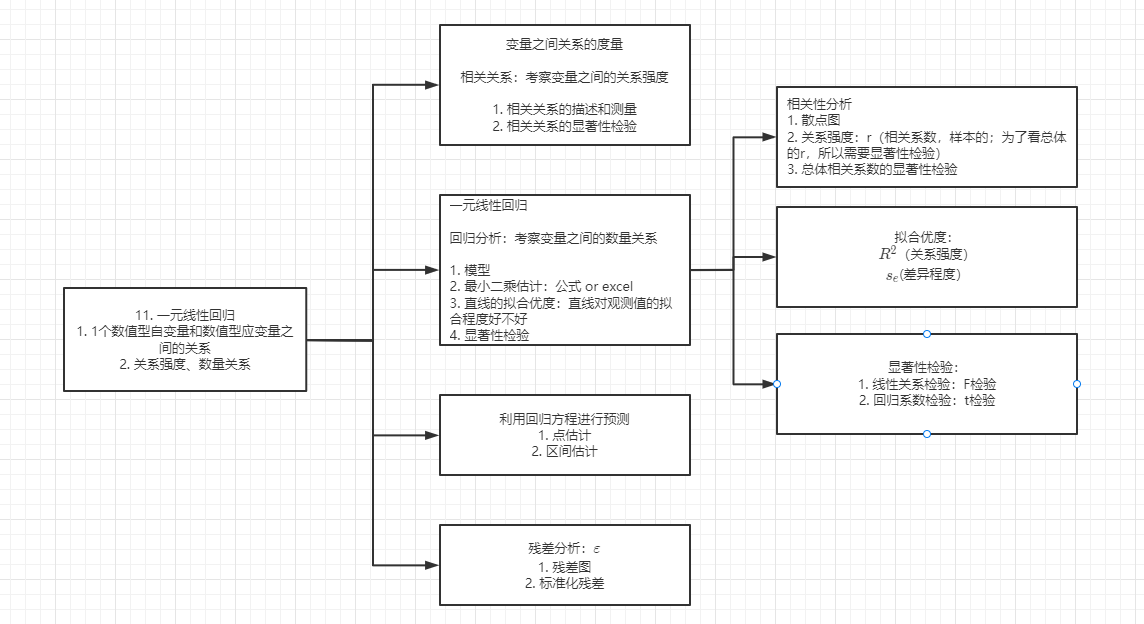展开全文线性回归 回归
• 之前提到过描述单个随机变量的一些工具，比如正针对整体总体细节的“分布”、针对总体概述的各种统计量（期望、方差等），也提到过针多元随机变量的描述量：协方差和相关系数，现在此总结下多个变量之间关系的研究。...

### 目录

之前提到过描述单个随机变量的一些工具，比如正针对整体总体细节的“分布”、针对总体概述的各种统计量（期望、方差等），也提到过针多元随机变量的描述量：协方差和相关系数，现在此总结下多个变量之间关系的研究。

注：针对机器学习的问题，“变量”可以直接理解为“特征”。

# 1. 散点图

研究两个变量之间关系的最简单方法是散点图（scatter plot）。但好的散点图的绘制并不简单。

注：可以将数据进行抖动（jittering），即加入随机噪音弥补四舍五入的效果，以减少丢失信息对散点图的影响。
但是，抖动数据通常只应用于视觉效果，你应该避免在分析时使用经过抖动处理的数据。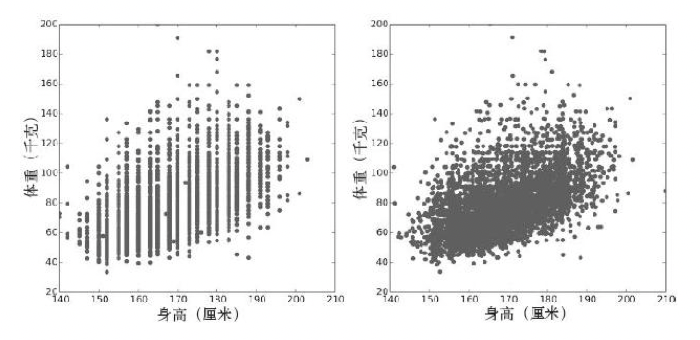即便经过了抖动处理，散点图也不是展示数据的最佳方法。图中有很多重叠的点，遮盖了密集部分的数据，使离群值显得特别突出。这种效果称为饱和（saturation）。

# 2. 描述关系特征

散点图能让我们对变量关系有个大体了解，而其他可视化方法则可以让我们更深入地了解变量关系的本质。一种方法是对一个变量进行分区，绘制另一个变量的百分位数

# 3. 相关性分析

相关性（correlation）是一个统计量，用于量化两个变量之间关系的强弱

度量相关性的困难之处在于，我们需要比较的变量通常使用不同的单位。即便变量使用相同的单位，也可能来自不同的分布。

这些问题有两个常见的解决方法。

• 将每个值都转换为标准分数（standard score），即其偏离均值的标准差数。这种转换会产生“Pearson乘积矩相关系数”。
• 将每个值都转换为秩，即其在所有值的排序列表中的索引。这种转换会产生“Spearman秩相关系数”。

## 3.1 协方差

协方差（covariance）可以度量两个变量共同变化的趋势
对于随机变量X和Y，两者的协方差定义如下：
Cov[X,Y] = E[(X-μx)(Y-μy)]，（中括号只是一种约定俗成的表示手段）可以这样理解：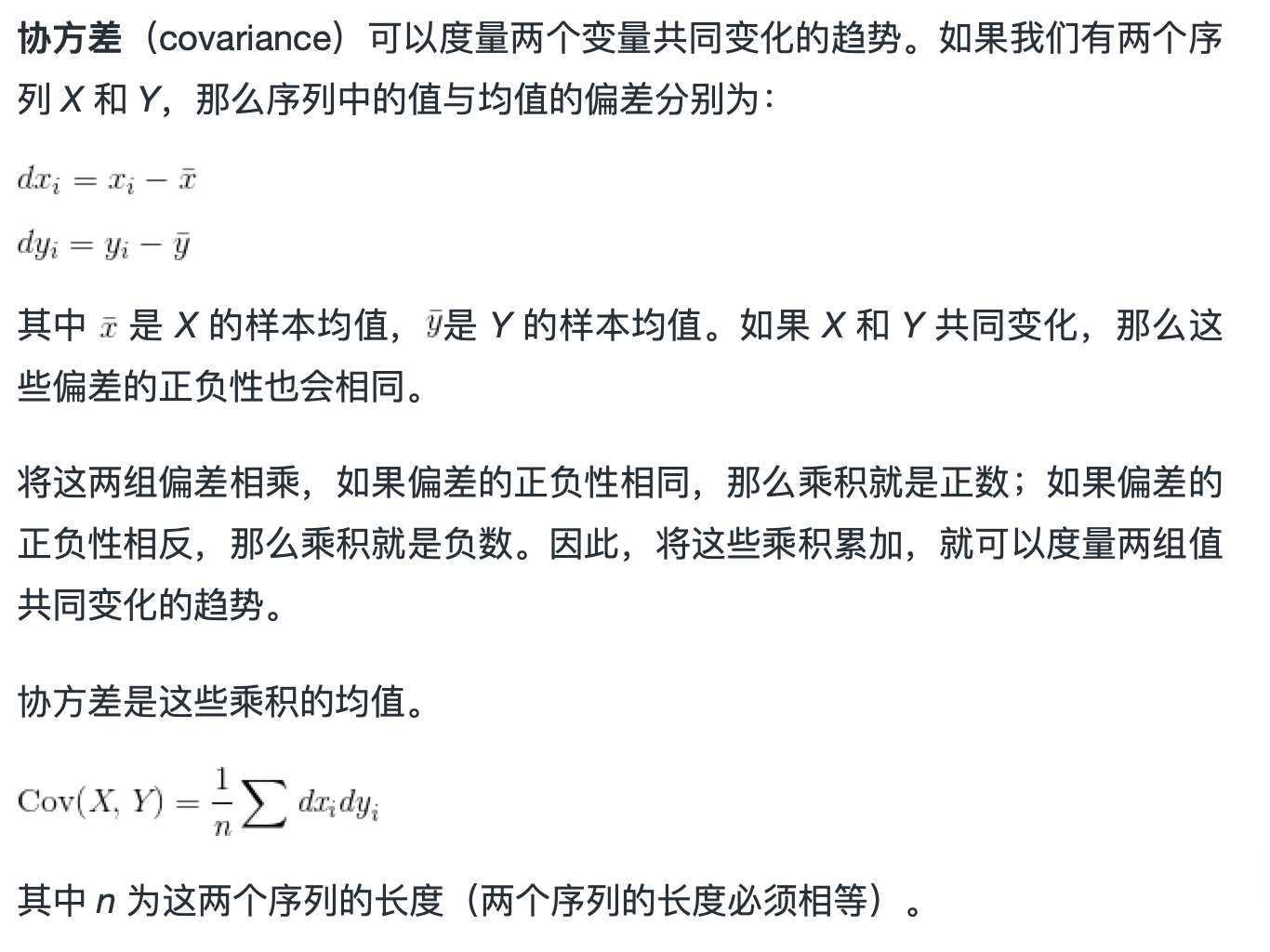• 协方差公式中包含的含义
协方差为正，表示X增大时，Y也增大——正相关性；
协方差为负，表示X增大时，Y倾向于减小——负相关性；
协方差为0时，表示X增大，Y没有明显的增大或减小的倾向——两者独立相关。

几点注意：

1.与方差的关系
Var[X] = E[(X-μ)2] = E[(X-μ)(X-μ)] ，其实方差就是一种特殊的协方差

2.协方差矩阵

我们可以引入一个协方差矩阵，将一组变量X1，X2，X3两两之间的协方差用矩阵的形式统一进行表达：

[
V[X~1~]      Cov[X~1~X~2~]      Cov[X~2~X~3~]
Cov[X~2~X~1~      V[X~2~]       Cov[X~2~X~3~]
Cov[X~2~X~1~]      Cov[X~3~X~2~]     V[X~3~]
]


注：看上面的公示就会发现若均值μ都为0 ，则计算会简便很多，所以一般先对变量进行0均值处理（xi或者yi减去他们的均值）

3.PCA降维的过程

假设我们研究的对象有两个特征属性X和Y，对 5 个样本进行数据采样的结果如下：

XY
样本122
样本226
样本346
样本488
样本548

我们的目标是对其降维，只用一维特征来表示每个样本，只用一维特征来表示每个样本。我们首先将其绘制在二维平面图中进行整体观察：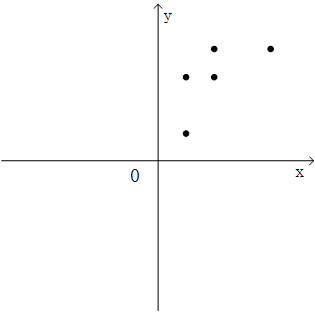查看这两个变量的协方差矩阵，

import numpy as np
import matplotlib.pyplot as plt

x = [2,2,4,8,4]
y = [2,6,6,8,8]
S = np.vstack((x,y))

print(np.cov(S))

[[ 6.  4.]
[ 4.  6.]]


结合之前的二维散点图可以发现5个样本的特征 X 和特征 Y 呈现出正相关性，数据彼此之间存在着影响。

若直接粗暴地去掉一个特征，可行么？则会变成：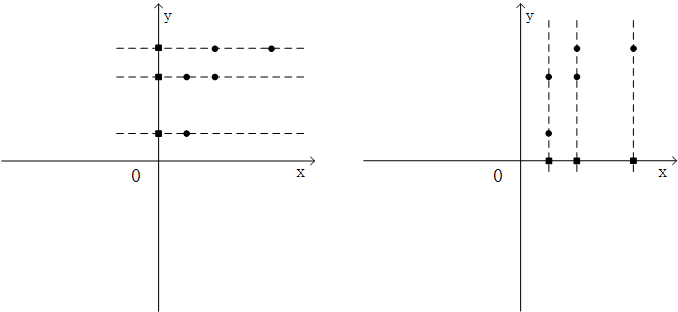显然效果不理想：忽视了数据中的内在结构关系，并且带来了非常明显的信息损失。
（降维——>高维数据向低维进行投影）

一个解决思路便是：

① 去除原始特征的相关性，使用心新的一组特征来表示原始数据
② 然后从新的彼此无关的特征中舍弃不重要的特征，保留较少的特征，实现降维。

首先，第一点的目的是使用新的特征来对样本来进行描述为了让这两个新特征满足彼此无关的要求，就需要让这两个新特征的协方差为0，构成的协方差矩阵是一个对角矩阵（原始特征X和Y的协方差不是0，只是一个普通的对称矩阵

对变量分别进行0均值处理后，通过求解协方差矩阵的特征向量，就可以得到线性无关的特征矩阵（图中两个新的坐标方向）。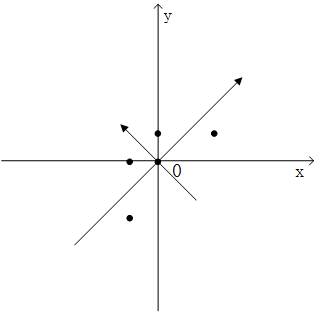接下来的工作就是从这两个特征中选取一个作为原始数据的特征表达，其判断标准是方差，方差越大表示这个特征里的数据分布的离散程度就越大，特征所包含的信息量就越大。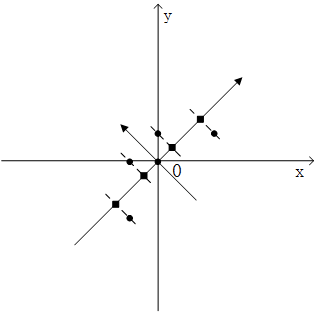# 3.2 Pearson相关性

协方差在一些计算中非常有用，但其含义很难解释，因此人们很少将协方差作为摘要统计量。别的不提，协方差的单位是 X 和 Y 的单位乘积，这一点就很难理解。例如，BRFSS数据集中体重和身高的协方差是113千克-厘米，天晓得这是什么意思。
解决这个问题的方法之一是将偏差除以标准差，得到标准分数，然后计算标准分数的乘积。
Pearson相关性容易计算，也易于解释。因为标准分数是无量纲（无单位），所以 ρ 也是无单位的。

# 非线性关系

如果Pearson相关性接近0，你可能会认为变量之间没有关系，但这个结论并不成立。Pearson相关性只度量了线性（linear）关系。如果变量之间存在非线性关系，那么 ρ 对变量相关性强弱的估计就可能是错误的。

# Spearman秩相关

如果变量之间的关系是线性的，而且变量大致符合正态分布，那么Pearson相关性能够很好地说明相关性的强弱。但是离群值会影响Pearson相关性的稳健性。Spearman秩相关能够缓解离群值以及偏斜分布的的影响，也可以用于描述变量的相关性。要计算Spearman相关性，必须计算每个值的秩（rank），即该值在排序样本中的索引。

# 相关性和因果关系

记住：“相关性并不意味着因果关系”

我们可以用 X 的信息，去预测 Y 的分布或者某些特征，但并不能告诉我们 X 的变化一定会导致 Y 的变化。

统计关系，无论多么强、多么富有启示性，都不能确立因果关系。因果关系的思想必须来自于统计学之外，来源于一些理论或者其他方面
——Kendall & Stuart（1961）

参考：

展开全文#绘制普通矩阵散点图
plot(dataframe)
#绘制带有拟合直线，最佳拟合曲线和直方图的矩阵散点图
library(car)
attach(dataframe)
scatterplotMatrix(~var1+var2+var3+...,diagonal="histogram",gap=0.5)

#绘制气泡图
symbols(var1,var2,circles = var3/pi) #用气泡图表示三个变量之间的关系，其中用一个变量的大小表示气泡的大小

#雷达图
library(fmsb)
radarchart(example2_5,axistype =0,seg = 4,maxmin=FALSE,vlabels = names(example2_5),plwd=2)
#第二行必须是最小值，vlabels变量的名称)
legend(x="topleft",legend = rownames(example2_5),col = 1:7,lwd=2,text.width = 0.5,cex = 0.6)

#平行坐标图（多线图）
outplot<-function(data){
nc<-ncol(data) #data的列数
nr<-nrow(data) #data的行数
plot(x=1:nc,ylim = c(min(data),max(data)),xaxt="n",type = "n",ylab = "值",cex.axis=0.6)
#xaxt="n" 设置x-轴但不显示，type = "n" 不作图
for(i in 1:nr){
lines(as.numeric(data[i,]),col=i,lwd=2,type="o")
}
legend(x="top",legend = rownames(data),col=1:nr,lwd=2,text.width = 1,cex=0.6)
axis(side=1,at=1:nc,labels =names(data),cex.axis=0.7 )
}
outplot(example2_5)
展开全文R
• 0.001），财政收入与城乡居民储蓄存款年底余额之间关系最为密切（r = 0.995，ρ < 0.001） 相关系数表明了各变量与财政收入之间的线性关系程度相当高，由此可以认为所选取的九个因素都与财政收入存在着线性关系...
• 如果能从一个变量的信息中得到另一个变量的信息，那么这两个变量之间就是相关的。
• python数据可视化seaborn（三）——探索变量之间关系 我们常常想知道变量之间是否存在关联，以及这些关联是否收到其他变量影响。可视化能够帮助我们非常直观的展示这些。 import numpy as np import pandas as pd ...python seaborn
• 回归模型+自变量和因变量之间关系、回归模型的种类、回归模型的输出类型、个数角度 目录 回归模型+自变量和因变量之间关系、回归模型的种类、回归模型的输出类型、个数角度 自变量和因变量之间关系 回归...机器学习 人工智能 大数据 数据挖掘 深度学习
• 变量之间关系图（1）散点图（2）指定分类变量的散点图使用调色板使用不同的形状（3）改变对角图使用KDE使用回归（4）改变点形状，使用参数，使用edgecolor 1.导入需要用的包 #-*- coding:utf-8 -*- from __...python java 大数据 javascript oracle
• 案例背景或目标：激素水平是否在对照组和实验组之间存在差异 分析方法：Bootstrap抽样，秩和检验，秩变换方法，cox回归 字段包括：性别，年龄，萎缩程度，胃粘膜细胞肠化生程度 基本思路：控制变量法，首先排除...
• 接着用图形的方式研究变量之间关系： pairs(iris) # 绘制任意两个矩阵之间的散点图，发现变量之间的相关性 四、看看其他方面 三维散点图： library(scatterplot3d) scatterplot...r语言
• 利用协方差，皮尔逊相关系数和斯皮尔曼相关系数确定变量间的关系 ...数据集中的变量之间可能存在复杂且未知的关系。重要的是发现和量化数据集的变量相关的程度。...在本教程中，你会了解到相关性是变量之间关系的统...
• 当数据存储为data.frame格式时，我们通常先将其转为可以使用 PerformanceAnalytics 包之间求相关，并将结果可视化。 chart.Correlation(dda, histogram=TRUE, pch=19) 其中，dda 为data.frame格式的数据，要求...
• 6 散点矩阵是很好的可视化变量之间关系的方法：能绘制散点图矩阵的函数paris(),cars包中的scatterplot Matrix(),以及gparis 包中的gpairs() 7 当变量高度有偏时，可使用设置plot()函数中的log选项对相应的坐标...
• 前言我们在分析两组变量之间的相关性时，比如X=[X1,X2,...,Xm]X=[X_1,X_2,...,X_m]和Y=[Y1,Y2,...,Yn]Y=[Y_1,Y_2,...,Y_n],最原始的方法就是直接计算X和Y的协方差矩阵，矩阵有m*n个值。有了协方差矩阵就得到了两两...机器学习 CCA 协方差
• 但我们可以通过IBM SPSS Statistics（win）中的交叉表功能来确定两个变量之间的关联是否存在。 一、录入数据 消费者的年龄与消费者的购买意愿是否存在关联？相信这是一个多数人都会感兴趣的问题。本文将以一组年龄...机器学习 r语言 人工智能
• 相关分析在数据量和维度较少时可用于数据初步探索，但对于数据量过于庞大（实际中数据维度通常也较大），可跳过此阶段，采用决策树模型对变量进行探索。机器学习
• 关系强度定义： 变量类型 定类变量：根据定性的原则区分各类别的变量 特点： 举例：性别、民族、婚姻状况 定序变量：等级次序 特点： 举例：文化程度、工厂规模、年龄大小 定距变量：区别同一类别中等级次序及其距离...
• 协方差衡量的是两个变量之间的相关性，如： 正相关：两个变量具有相同的变化趋势（也称同方向），要么同时变大，要么同时变小，这时协方差是正的； 负相关：两个变量具有相反的变化趋势（也称反方向），一个变...协方差 数学
• 站在2022的第一天想说点...但是，在日常数据分析实践中，由于数据源的特征分布情况，特征相关性分析往往是针对连续变量与连续变量，如pearson相关系数等，而对分类变量的相关性较少关注。本文基于SAS实操，介绍下不同类数据挖掘 机器学习
• 变量线性相关分析 现实世界中的数据科学 (Data Science in the Real World) This article aims to present two ways of calculating non linear correlation between any number of discrete variables. The ...python java 机器学习 leetcode 数据结构
• 一 用表格方式汇总两个变量的数据 1 交叉分组表 常用于一个变量为分类型变量，一个变量为数量型变量 下面是由洛杉矶300家饭店组成的一个样本，其质量等级与参加数据的应用。 质量等级是一个分类变量，等级...数据挖掘 机器学习
• 原文链接：http://tecdat.cn/?p=18169 比如说分类变量为是否幸存、是因变量，连续变量为年龄、是自变量，这两者可以做相关分析吗？两者又是否可以做回归分析？ 我们考虑泰坦尼克号数据集，R语言 逻辑回归
• 如何在Excel中计算两个变量之间的相关系数？我们通常使用相关系数(介于-1和1之间的值)来显示两个变量之间的相关程度。 在Excel中，我们还可以使用CORREL函数来查找两个变量之间的相关系数。请注意：相关系数+1表示...
• 典型相关分析（Canonical correlation analysis）（二）：原始变量与典型变量之间的相关性 、典型相关系数的检验 典型相关分析（Canonical correlation analysis）（三）： 职业满意度典型相关分析案例......